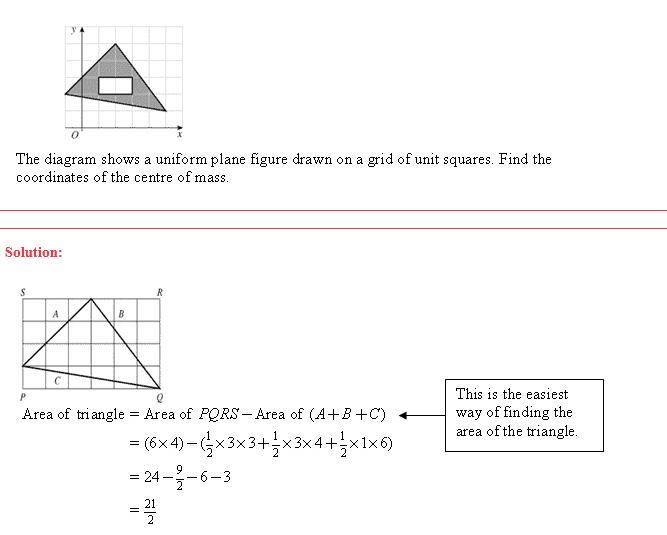# Area of triangle

phospho
Could anyone tell me how they found the area of the triangle?When I try to use 0.5*b*h I get 10.64483443, not 10.5

Homework Helper
Gold Member
They subtracted the areas of the three right triangles outside the given triangle from the area of the rectangle.

How did you calculate b and h for the triangle? I'm guessing you did it wrong.

phospho
They subtracted the areas of the three right triangles outside the given triangle from the area of the rectangle.

How did you calculate b and h for the triangle? I'm guessing you did it wrong.

I found the midpoint of the two bottom verticies of the triangle, then found the length from that midpoint to the top vertex and used that as the height. The length of the two bottom vertices I used as the base.

I see what they done now, thanks, but is how could I have done using the b*h*0.5?

Homework Helper
Gold Member
I found the midpoint of the two bottom verticies of the triangle, then found the length from that midpoint to the top vertex and used that as the height. The length of the two bottom vertices I used as the base.

I see what they done now, thanks, but is how could I have done using the b*h*0.5?

You mean "did".

The line from the midpoint of a side to the opposite vertex is a median. Medians are not generally perpendicular to the side, so its length is not the height of the triangle.

To do it that way you would have to find where the line from the vertex perpendicular to the other side intersects the other side and use that line (called the altitude) for the height.

phospho
You mean "did".

The line from the midpoint of a side to the opposite vertex is a median. Medians are not generally perpendicular to the side, so its length is not the height of the triangle.

To do it that way you would have to find where the line from the vertex perpendicular to the other side intersects the other side and use that line (called the altitude) for the height.